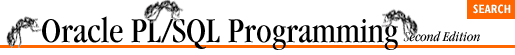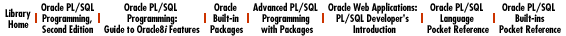home | O'Reilly's CD bookshelfs | FreeBSD | Linux | Cisco | Cisco Exam

### 10.6 Filling the Rows of a PL/SQL Table

You can assign values to rows of a table in several ways:

• Direct assignment

• Iterative assignment

• Aggregate assignment

These methods are described in the following sections.

### 10.6.1 Direct Assignment

As shown in previous examples, you can simply assign a value to a row with the assignment operator:

```countdown_test_list (43) := 'Internal pressure';
company_names_table (last_name_row) := 'Johnstone Clingers';```

Direct assignment makes sense when you need to make a change to a specific row. But what do you use when you want to fill a whole set of rows, for example, unloading a whole cursor-full of information from a database table? Here, iterative assignment may be more appropriate.

### 10.6.2 Iterative Assignment

In order to fill up multiple rows of a table, I recommend taking advantage of a PL/SQL loop. Within the loop you will still perform direct assignments to set the values of each row, but the primary key value will be set by the loop rather than hardcoded into the assignment itself.

In the following example, I use a WHILE loop to fill and then display a PL/SQL date table with the next set of business days, as specified by the ndays_in parameter:

```/* Filename on companion disk: bizdays.sp */
CREATE OR REPLACE PROCEDURE show_bizdays
(start_date_in IN DATE := SYSDATE, ndays_in IN INTEGER := 30)
IS
TYPE date_tabtype IS TABLE OF DATE INDEX BY BINARY_INTEGER;
bizdays date_tabtype;

/* The row in the table containing the nth_day */
nth_day  BINARY_INTEGER := 1;
v_date DATE := start_date_in;
BEGIN
/* Loop through the calendar until enough biz days are found */
WHILE nth_day <= ndays_in
LOOP
/* If the day is not on the weekend, add to the table. */
IF TO_CHAR (v_date, 'DY') NOT IN ('SAT', 'SUN')
THEN
bizdays (nth_day) := v_date;
DBMS_OUTPUT.PUT_LINE (v_date);
nth_day := nth_day + 1;
END IF;
v_date := v_date + 1;
END LOOP;
END show_bizdays;
/```

As you can see from this example, using the WHILE loop produces a neat, sequential load of the PL/SQL table.

### 10.6.3 Aggregate Assignment

Just as you can assign one entire record to another record of the same type and structure, you can perform aggregate assignments with tables as well. In order to transfer the values of one table to another, the datatype of the two tables must be compatible. Beyond that you simply use the assignment operator ( :=) to transfer the values of one table to the other. The following example contains an example of an aggregate table assignment:

```DECLARE
TYPE name_table IS TABLE OF VARCHAR2(100) INDEX BY BINARY_INTEGER;
old_names name_table;
new_names name_table;
BEGIN
/* Assign values to old_names table */
old_names(1) := 'Smith';
old_names(2) := 'Harrison';

/* Assign values to new_names table */
new_names(111) := 'Hanrahan';
new_names(342) := 'Blimey';

/* Transfer values from new to old */
old_names := new_names;

/* This assignment will raise NO_DATA_FOUND */
DBMS_OUTPUT.PUT_LINE (old_names (1));
END;```

A table-level assignment completely replaces the previously defined rows in the table. In the preceding example, rows 1 and 2 in old_names are defined before the last, aggregate assignment.

After the assignment, only rows 111 and 342 in the old_names table have values.10.5 Referencing and Modifying PL/SQL Table Rows10.7 Clearing the PL/SQL Table